In addition, to the option to run a significance test between data points in the same series in a chart or table, you can also run significance tests between series.

The setting for Series is the highlighted items in the image below.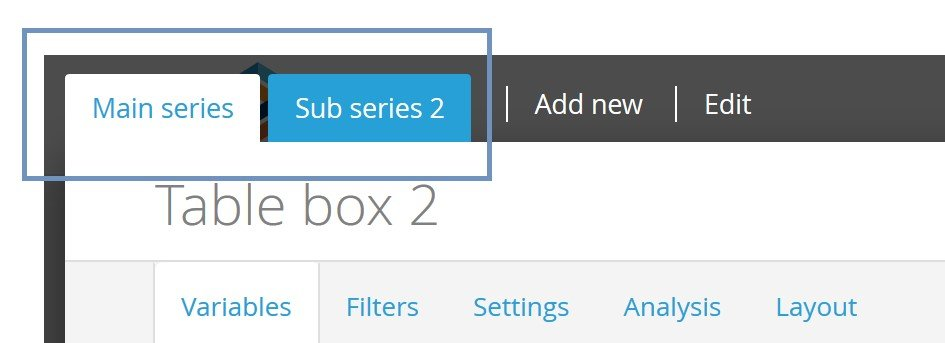Since the structure of data can be completely different between series, then a cell by cell comparison is used when running significance test between series. This means that cell A1 in series 1 is compared to cell A1 in series 2, and cell A2 in series 1 is compared to cell A2 in series 2, and so on and so forth. Below is an example of a table that tests different series against each other.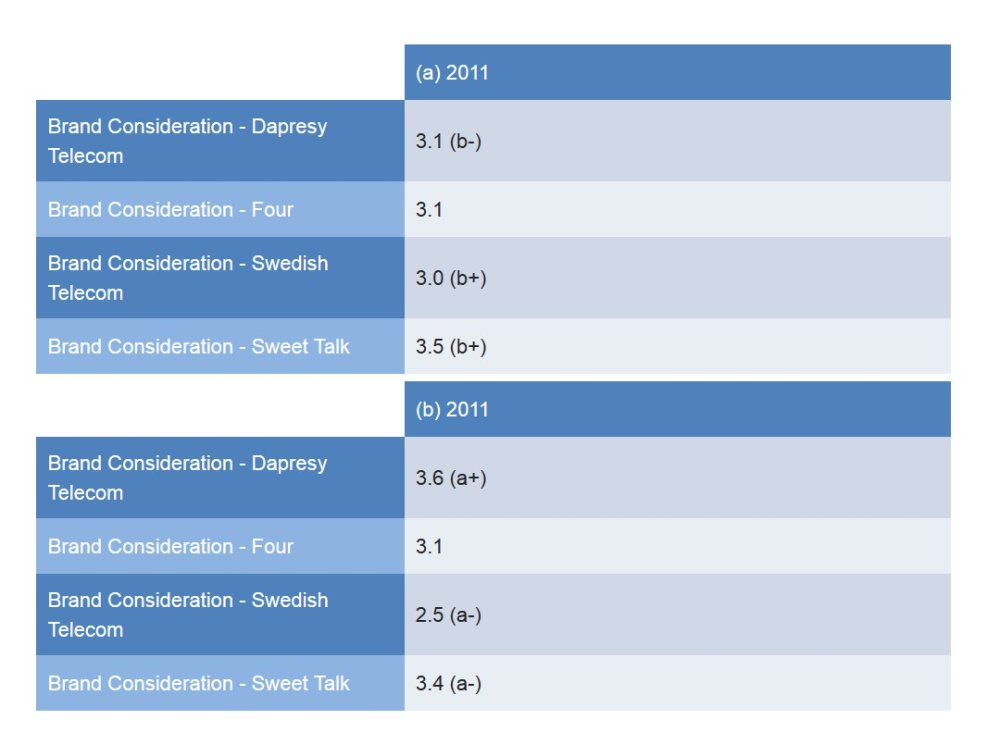If a significance test is going to be run between two or more series, then all series have to use the same calculation type. Either all series need to be “Mean –Categorical questions” or “Percentage share – categorical questions.”

To setup a significance test between two or more series, just follow these two steps:

• In the section “Test between,” select the “Series” option (see image below).
• This option is only available in the Main series. It is also only available if the chart/table has multiple series and if all series are either “Mean –Categorical questions” or “Percentage share – categorical questions”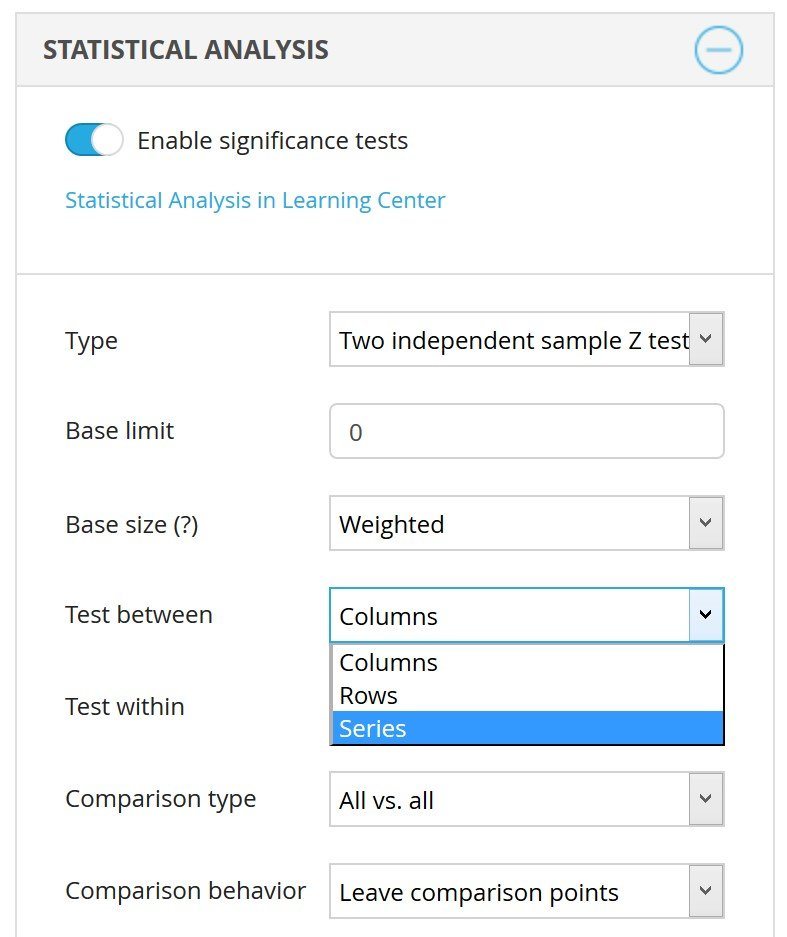• Select how the series will be compared to each other in the “Comparison type” list. The same options that you use when doing a regular significance test are available:
• All vs. all
• Previous data point
• Next data point
• First data point
• Last data point

Below you can see an example of each “Comparison type” option. The examples are based on a chart containing three series: Series 1, Series 2 and Series 3.

Example 1: All Vs All

• Cell A1 in series 1 is compared to A1 in series 2 and vice versa
• Cell A1 in series 1 is compared to A1 in series 3 and vice versa
• Cell A1 in series 2 is compared to A1 in series 2 and vice versa

Example 2: Previous data point

• Cell A1 in series 2 is compared to A1 in series 1
• Cell A1 in series 3 is compared to A1 in series 2

Example 3: Next data point

• Cell A1 in series 1 is compared to A1 in series 2
• Cell A1 in series 2 is compared to A1 in series 3

Example 4: First data point

• Cell A1 in series 2 is compared to A1 in series 1
• Cell A1 in series 3 is compared to A1 in series 1

Example 5: Last data point

• Cell A1 in Series 1 is compared to A1 Series 3
• Cell A1 in Series 2 is compared to A1 Series 3

When running a significance test between series, the value comparison is done cell by cell. A1 in series 1 is compared to A1 in series 2, and A2 in series 1 is compared to A2 in series 2, and so on and so forth.
In a situation where one of the series has fewer cells than the other series, then the test will not run in the cells that don’t have a corresponding cell in the comparison series.

See examples below.

Example 1: All cells in series 1 have a corresponding cell in series 2. The yellow cell in series 1 is tested against the yellow cell in series 2. The green cell in series 1 is tested against the green cell in series 2, and so on.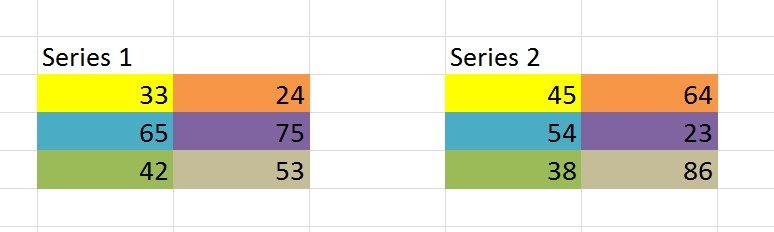Example 2: The cell B2 in Series 1 has no value. Therefore, no test is applied to cell B2 in Series 2.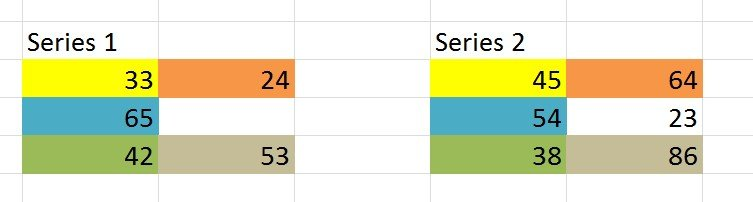Example 3: The series 1 has 3 rows of data while series 2 only has 2 rows of data. No test is applied to the values in row 3 in series 1.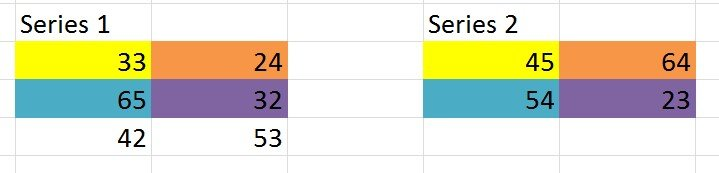### What to compare between Series?

Since the structure of data can be completely different between series, the comparison can be done by the cell index (cell A1 in series 1 is compared to cell A1 in series 2, and cell A2 in series 1 is compared to cell A2 in series 2, and so on and so forth) or by using a text match between the items in the different series. Below you see use cases of both the cell index and the text match comparison.

Here we see a basic example where the cell index has to be used as comparison logic for benchmarking. In this example the main series shows the top of mind and the in mind awareness for Dapresy Telecom and the sub series shows their target values (the target values are stored in numeric variables in this example). As no text match can be made between the series a cell index comparisons is applied, hence to that it is very important that the order of the items in both series is the same so the right values are compared to each other (cell A1 in series 1 is compared to cell A1 in series 2, and cell A2 in series 1 is compared to cell A2 in series 2). If we would want to apply significance testing here, we would need to compare series by Index.

Text match in rows has to be used as comparison logic when the text is the same, but the positions are different. In this example the main series shows the top of mind awareness for the latest month, the sub series shows the same awareness result but for the year to date. The brands are ordered by value so a cell index comparison cannot be applied as the brands have different positions.You can also select to test all values in the main series against a single value in the sub-series.

Here we see the option that appears when running a statistical test between series in Storyteller charts and tables.